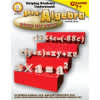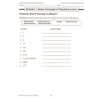••# Helping Students Understand Pre-Algebra Step By Step

Product Number: CD-404021
Facilitate a smooth transition from arithmetic to pre-algebra for students in grades 7 and up using Helping Students Understa...
Quantity

Facilitate a smooth transition from arithmetic to pre-algebra for students in grades 7 and up using Helping Students Understand Pre-Algebra.

This 128-page math book includes step-by-step instructions with these resources:

• examples
• practice problems using the concepts
• real-life applications
• a list of symbols and terms
• tips

The book supports NCTM standards and includes chapters on topics such as:

• basic number concepts
• operations and variables
• integers
• exponents
• square roots
• patterns

Features: